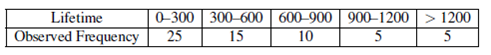# (Solved): The lifetimes of 60-watt filament light bulbs are thought to be exponentially distributed. One hundr ...

The lifetimes of 60-watt filament light bulbs are thought to be exponentially distributed. One hundred such light bulbs were tested by the manufacturer, resulting in the following grouped lifetime data.Test, at α = :05, the hypothesis that light bulbs of this type are exponentially distributed. Assume that, under the assumption of exponentiality, the MLE of the mean lifetime calculated from the sample of 100 bulbs was found to be 500 hours.

\begin{tabular}{|c|c|c|c|c|c|} \hline Lifetime & & & & & \\ \hline Observed Frequency & 25 & 15 & 10 & 5 & 5 \\ \hline \end{tabular}

We have an Answer from Expert